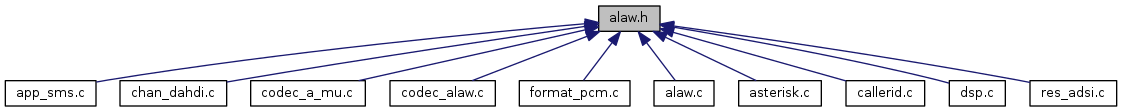Asterisk - The Open Source Telephony Project  18.5.0
alaw.h File Reference

A-Law to Signed linear conversion. More...

This graph shows which files directly or indirectly include this file:Go to the source code of this file.

## Macros

#define AST_ALAW(a)   (__ast_alaw[(int)(a)])

#define AST_ALAW_BIT_LOSS   4

#define AST_ALAW_SIGN_BIT   0x80

#define AST_ALAW_STEP   (1 << AST_ALAW_BIT_LOSS)

#define AST_ALAW_TAB_SIZE   (32768 / AST_ALAW_STEP + 1)

#define AST_LIN2A(a)   (__ast_lin2a[((unsigned short)(a)) >> 3])

## Functions

void ast_alaw_init (void)
To init the alaw to slinear conversion stuff, this needs to be run. More...

## Variables

short __ast_alaw 

unsigned char __ast_lin2a 
converts signed linear to alaw More...

## Detailed Description

A-Law to Signed linear conversion.

Definition in file alaw.h.

## ◆ AST_ALAW

 #define AST_ALAW ( a ) (__ast_alaw[(int)(a)])

Definition at line 84 of file alaw.h.

Definition at line 36 of file alaw.h.

Referenced by alaw2linear(), and linear2alaw().

## ◆ AST_ALAW_BIT_LOSS

 #define AST_ALAW_BIT_LOSS   4

Definition at line 32 of file alaw.h.

## ◆ AST_ALAW_SIGN_BIT

 #define AST_ALAW_SIGN_BIT   0x80

Definition at line 35 of file alaw.h.

## ◆ AST_ALAW_STEP

 #define AST_ALAW_STEP   (1 << AST_ALAW_BIT_LOSS)

Definition at line 33 of file alaw.h.

Referenced by ast_alaw_init().

## ◆ AST_ALAW_TAB_SIZE

 #define AST_ALAW_TAB_SIZE   (32768 / AST_ALAW_STEP + 1)

Definition at line 34 of file alaw.h.

## ◆ AST_LIN2A

 #define AST_LIN2A ( a ) (__ast_lin2a[((unsigned short)(a)) >> 3])

Definition at line 50 of file alaw.h.

## ◆ ast_alaw_init()

 void ast_alaw_init ( void )

To init the alaw to slinear conversion stuff, this needs to be run.

Definition at line 152 of file alaw.c.

Referenced by asterisk_daemon().

153 {
154  int i;
155  /*
156  * Set up mu-law conversion table
157  */
158 #ifndef G711_NEW_ALGORITHM
159  for (i = 0; i < 256; i++) {
160  __ast_alaw[i] = alaw2linear(i);
161  }
162  /* set up the reverse (mu-law) conversion table */
163  for (i = -32768; i < 32768; i++) {
164  __ast_lin2a[((unsigned short)i) >> 3] = linear2alaw(i);
165  }
166 #else
167  for (i = 0; i < 256; i++) {
168  __ast_alaw[i] = alaw2linear(i);
169  }
170  /* set up the reverse (a-law) conversion table */
171  for (i = 0; i <= 32768; i += AST_ALAW_STEP) {
172  AST_LIN2A_LOOKUP(i) = linear2alaw(i, 0 /* half-cooked */);
173  }
174 #endif
175
176 #ifdef TEST_CODING_TABLES
177  for (i = -32768; i < 32768; ++i) {
178 #ifndef G711_NEW_ALGORITHM
179  unsigned char e1 = linear2alaw(i);
180 #else
181  unsigned char e1 = linear2alaw(i, 1);
182 #endif
183  short d1 = alaw2linear(e1);
184  unsigned char e2 = AST_LIN2A(i);
185  short d2 = alaw2linear(e2);
186  short d3 = AST_ALAW(e1);
187
188  if (e1 != e2 || d1 != d3 || d2 != d3) {
189  ast_log(LOG_WARNING, "a-Law coding tables test failed on %d: e1=%u, e2=%u, d1=%d, d2=%d\n",
190  i, (unsigned)e1, (unsigned)e2, (int)d1, (int)d2);
191  }
192  }
193  ast_log(LOG_NOTICE, "a-Law coding tables test complete.\n");
194 #endif /* TEST_CODING_TABLES */
195
196 #ifdef TEST_TANDEM_TRANSCODING
197  /* tandem transcoding test */
198  for (i = -32768; i < 32768; ++i) {
199  unsigned char e1 = AST_LIN2A(i);
200  short d1 = AST_ALAW(e1);
201  unsigned char e2 = AST_LIN2A(d1);
202  short d2 = AST_ALAW(e2);
203  unsigned char e3 = AST_LIN2A(d2);
204  short d3 = AST_ALAW(e3);
205
206  if (e1 != e2 || e2 != e3 || d1 != d2 || d2 != d3) {
207  ast_log(LOG_WARNING, "a-Law tandem transcoding test failed on %d: e1=%u, e2=%u, d1=%d, d2=%d, d3=%d\n",
208  i, (unsigned)e1, (unsigned)e2, (int)d1, (int)d2, (int)d3);
209  }
210  }
211  ast_log(LOG_NOTICE, "a-Law tandem transcoding test complete.\n");
212 #endif /* TEST_TANDEM_TRANSCODING */
213
214 }
unsigned char __ast_lin2a
converts signed linear to alaw
Definition: alaw.c:146
short __ast_alaw
Definition: alaw.c:150
#define LOG_WARNING
Definition: logger.h:274
#define AST_ALAW(a)
Definition: alaw.h:84
#define ast_log
Definition: astobj2.c:42
#define AST_ALAW_STEP
Definition: alaw.h:33
static short int alaw2linear(unsigned char alaw)
Definition: alaw.c:112
#define LOG_NOTICE
Definition: logger.h:263
static unsigned char linear2alaw(short int linear)
Definition: alaw.c:38
#define AST_LIN2A(a)
Definition: alaw.h:50

## ◆ __ast_alaw

 short __ast_alaw

help

Definition at line 150 of file alaw.c.

Referenced by ast_alaw_init().

## ◆ __ast_lin2a

 unsigned char __ast_lin2a

converts signed linear to alaw

Definition at line 146 of file alaw.c.

Referenced by ast_alaw_init().